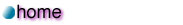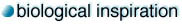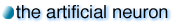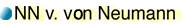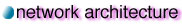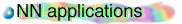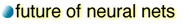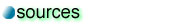The travelling salesman's problem:

Definition: Given a number of cities on a plane, find the shortest path through which one can visit all of the cities.

In contrast to using recursion to try all the different possibilities, we are going to approximate the solution using a Kohonen SOM, which organizes itself like a elastic rubber band. The basic idea of this solution is to start out with a elastic net in a random orientation:(Fig.1) A randon ring.

The algorithm is to choose a random city each time, and pull the point on the net that is closest to the city towards the city:(Fig.2) A point pulled towards Vashon Island brings the net closer to the city.

Since the net is elastic, each pull changes the shape of the net. After many pulls for different cities, the net eventually converges into a ring around the cities. Given the elastic property of the ring, the length of the ring tends to be minimized. This is how the TSP can be approximated.

The details:

As in simple competitive networks, the weight vectors of the networks are randomly assigned at the beginning in SOM's. Another similarity is that SOM also identifies the winner (the perceptron whose weight vector is closest to the input vector) every time an input vector is presented. The only difference is that while in simple competitive networks only the winner learns, in SOM's all nodes learn, but the rate of learning varies inversely with the node's physical distance from the winner.

The Kohonen SOM used in solving the TSP has the following structure:(Fig.3) A Kohonen net with a ring-shaped top layer.

Recall the elastic rubber-band model mentioned above, such a ring shaped map simulates a rubber-band if we consider the weight vectors as points on a plane. We can join these points together according to the position of their respective perceptron in the ring of the top layer of the network.

Suppose the coordinates of a city (x, y) is presented as the input vector of the network, the network will identify the weight vector closest to the city and move it and its neighbors closer to the city. In this manner, the weight vectors behave as points on a rubber band and each "learning" is analogous to pulling the closest point of the band towards a city. The rule that the amount of learning varies inversely with the physical distance between the node and the winner is what leads to the elastic property of the rubber band.